library(khroma)

## Introduction

Crameri (2018) offers carefully chosen schemes, ready for mapping data, with colours that are:

• Perceptually uniform,
• Perceptually ordered,
• Colour vision deficiency friendly,
• Readable as black and white print,
• Citable & reproducible.

All the scales presented in Crameri (2021) are implemented here, for use with base R graphics or ggplot2.

## Diverging data

### broc

broc <- colour("broc")
plot(broc(256))### cork

cork <- colour("cork")
plot(cork(256))### vik

vik <- colour("vik")
plot(vik(256))### lisbon

lisbon <- colour("lisbon")
plot(lisbon(256))### tofino

tofino <- colour("tofino")
plot(tofino(256))### berlin

berlin <- colour("berlin")
plot(berlin(256))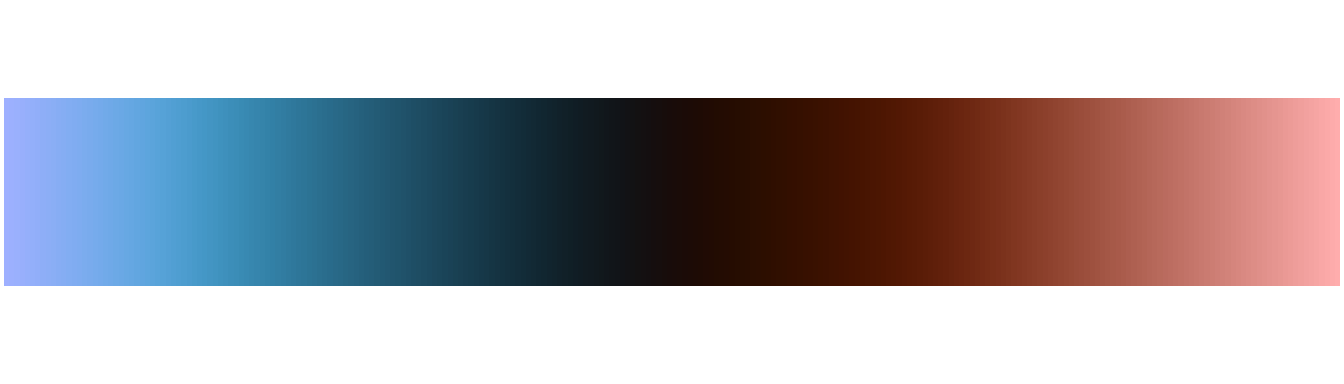### roma

roma <- colour("roma")
plot(roma(256))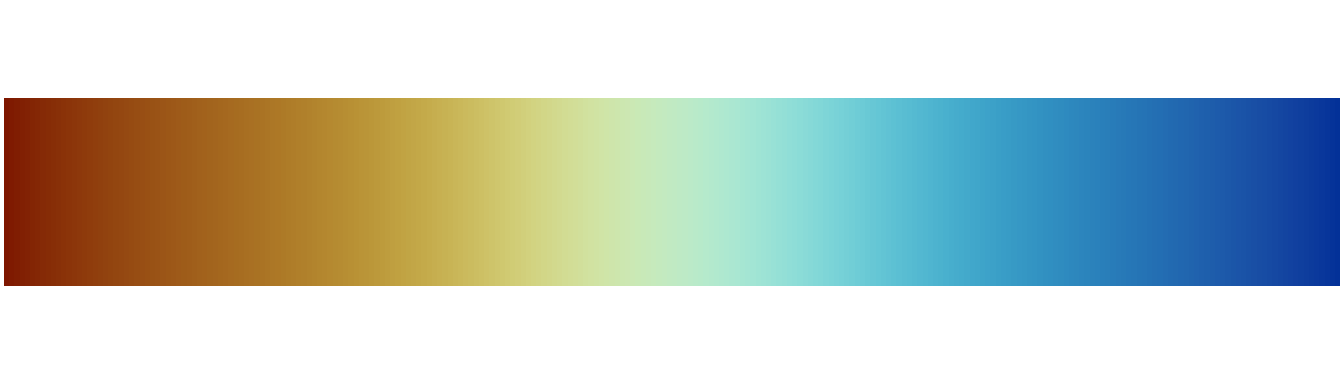### bam

bam <- colour("bam")
plot(bam(256))### vanimo

vanimo <- colour("vanimo")
plot(vanimo(256))## Sequential data

### batlow

batlow <- colour("batlow")
plot(batlow(256))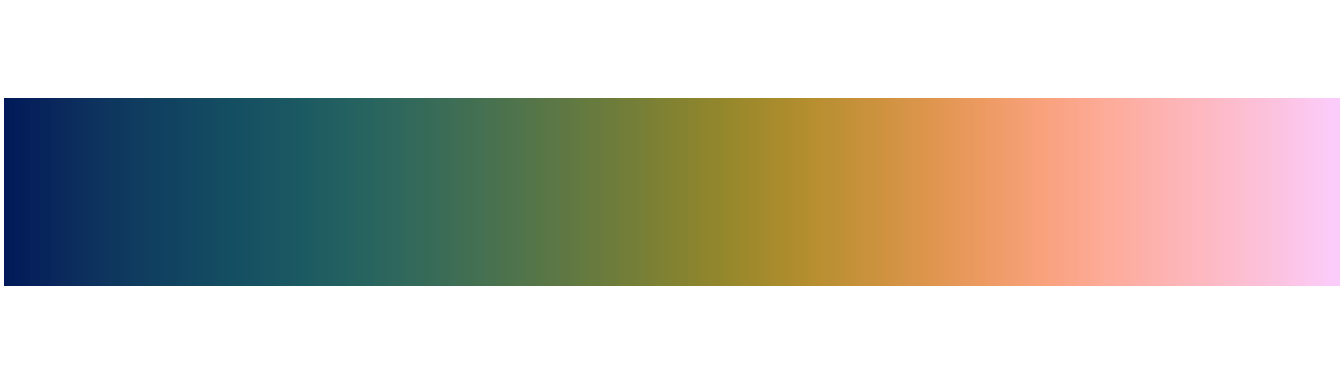### batlowW

batlowW <- colour("batlowW")
plot(batlowW(256))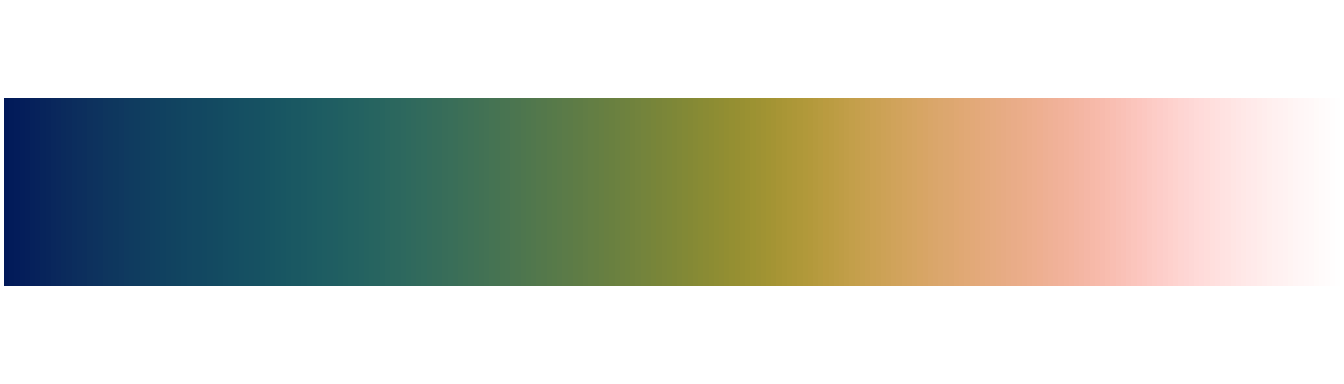### batlowK

batlowK <- colour("batlowK")
plot(batlowK(256))### devon

devon <- colour("devon")
plot(devon(256))### lajolla

lajolla <- colour("lajolla")
plot(lajolla(256))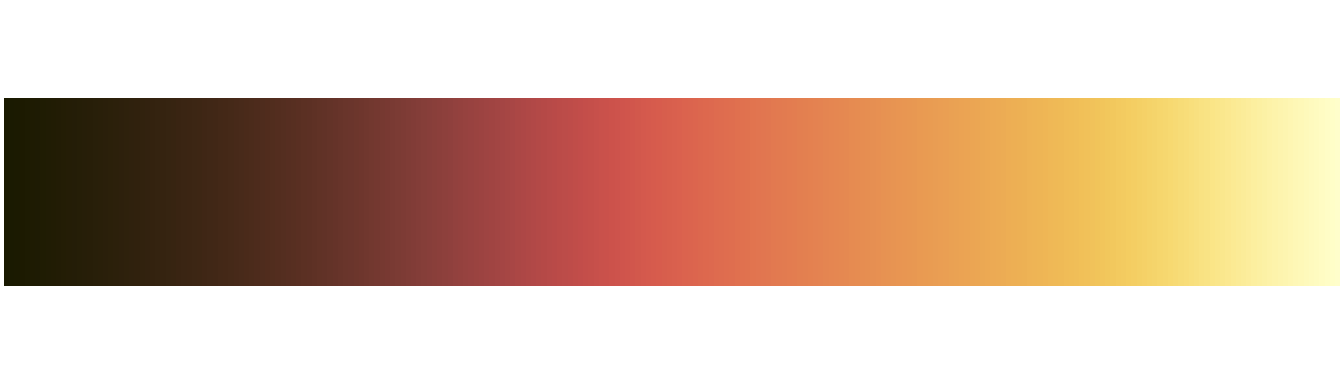### bamako

bamako <- colour("bamako")
plot(bamako(256))### davos

davos <- colour("davos")
plot(davos(256))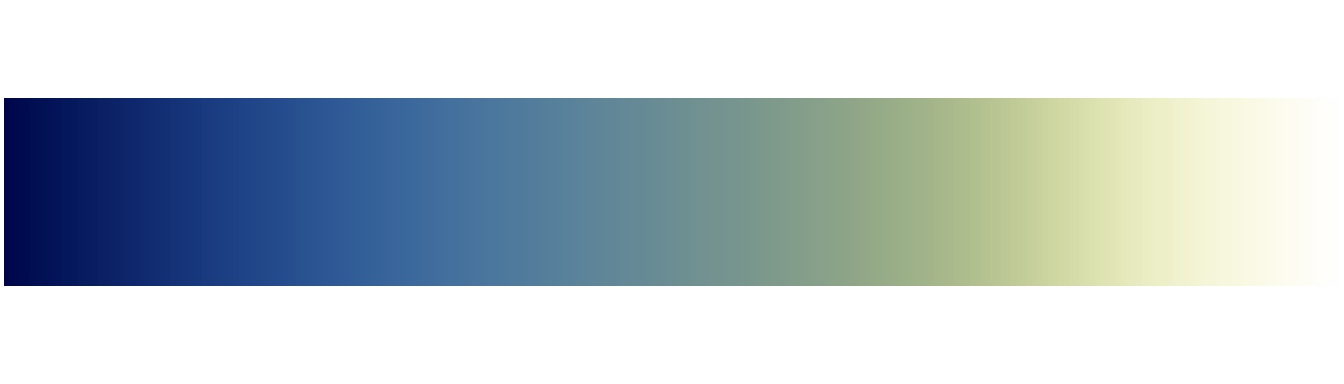### bilbao

bilbao <- colour("bilbao")
plot(bilbao(256))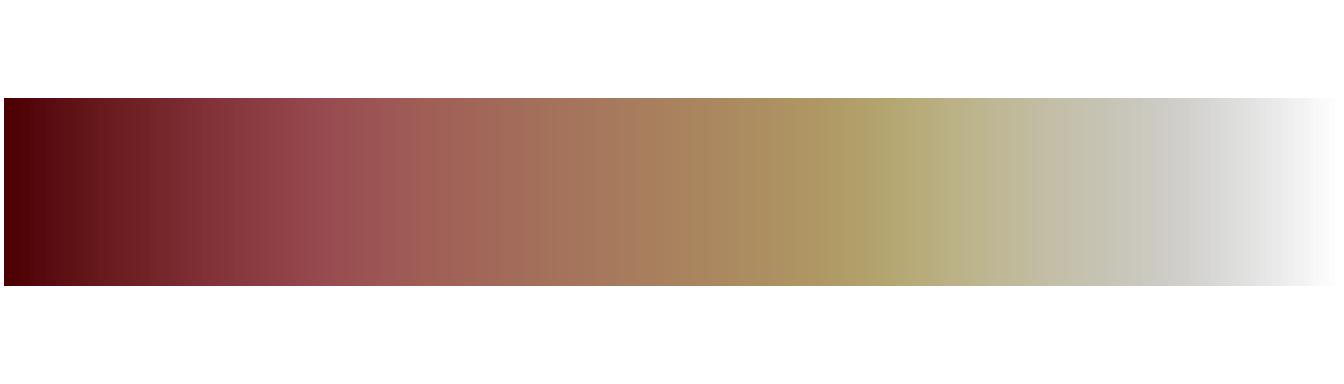### nuuk

nuuk <- colour("nuuk")
plot(nuuk(256))### oslo

oslo <- colour("oslo")
plot(oslo(256))### grayC

grayC <- colour("grayC")
plot(grayC(256))### hawaii

hawaii <- colour("hawaii")
plot(hawaii(256))### lapaz

lapaz <- colour("lapaz")
plot(lapaz(256))### tokyo

tokyo <- colour("tokyo")
plot(tokyo(256))### buda

buda <- colour("buda")
plot(buda(256))### acton

acton <- colour("acton")
plot(acton(256))### turku

turku <- colour("turku")
plot(turku(256))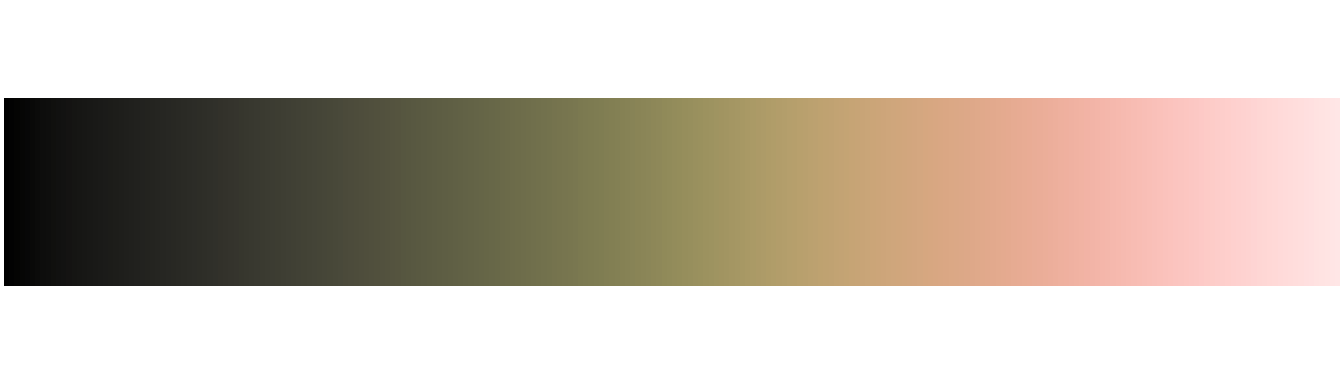### imola

imola <- colour("imola")
plot(imola(256))## Multi-sequential data

### oleron

oleron <- colour("oleron")
plot(oleron(256))### bukavu

bukavu <- colour("bukavu")
plot(bukavu(256))### fes

fes <- colour("fes")
plot(fes(256))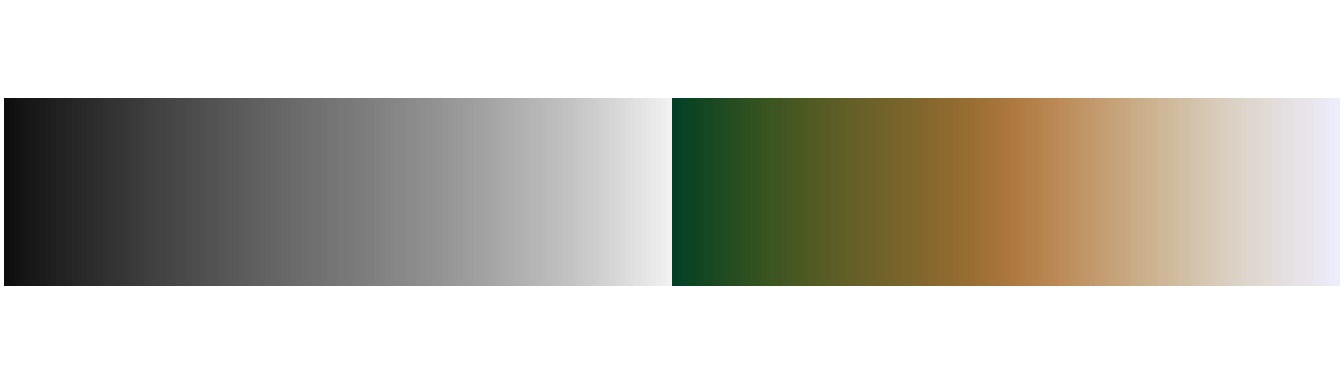Crameri, Fabio. 2018. “Geodynamic Diagnostics, Scientific Visualisation and StagLab 3.0.” Geoscientific Model Development 11 (6): 2541–62. https://doi.org/10.5194/gmd-11-2541-2018.
———. 2021. Scientific Colour Maps (version 7.0.0). Zenodo. https://doi.org/10.5281/zenodo.4491293.